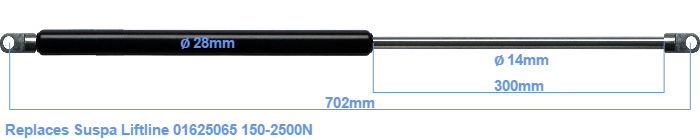# Replacement for Suspa Liftline 01625065 150-2500N

£50.98 (excl. VAT)

Replacement gas spring for the Suspa Liftline 01625065 150-2500 Newton. The eye has a thickness of 10mm and a hole diameter of 10.1mm. Brand: Hahn.

Please note:
The thickness of the eye of this replacement is 10mm instead of 12mm.
 Force Choose an option150 Newton175 Newton200 Newton225 Newton250 Newton275 Newton300 Newton325 Newton350 Newton375 Newton400 Newton425 Newton450 Newton475 Newton500 Newton525 Newton550 Newton575 Newton600 Newton625 Newton650 Newton675 Newton700 Newton725 Newton750 Newton775 Newton800 Newton825 Newton850 Newton875 Newton900 Newton925 Newton950 Newton975 Newton1000 Newton1025 Newton1050 Newton1075 Newton1100 Newton1125 Newton1150 Newton1175 Newton1200 Newton1225 Newton1250 Newton1275 Newton1300 Newton1325 Newton1350 Newton1375 Newton1400 Newton1425 Newton1450 Newton1475 Newton1500 Newton1525 Newton1550 Newton1575 Newton1600 Newton1625 Newton1650 Newton1675 Newton1700 Newton1725 Newton1750 Newton1775 Newton1800 Newton1825 Newton1850 Newton1875 Newton1900 Newton1925 Newton1950 Newton1975 Newton2000 Newton2025 Newton2050 Newton2075 Newton2100 Newton2125 Newton2150 Newton2175 Newton2200 Newton2225 Newton2250 Newton2275 Newton2300 Newton2325 Newton2350 Newton2375 Newton2400 Newton2425 Newton2450 Newton2475 Newton2500 NewtonClear
This gas spring is also known as 16-6 016 25065, 16-6-368-300-A31-B31.